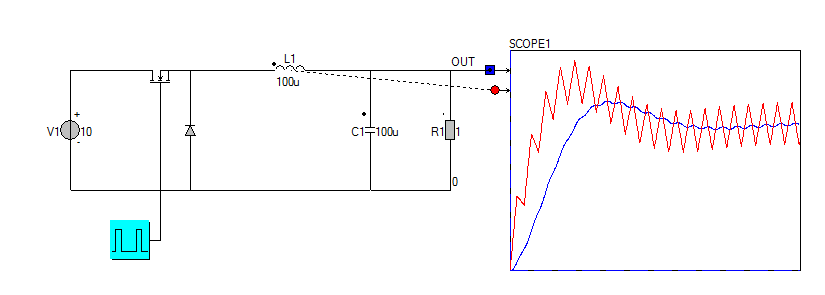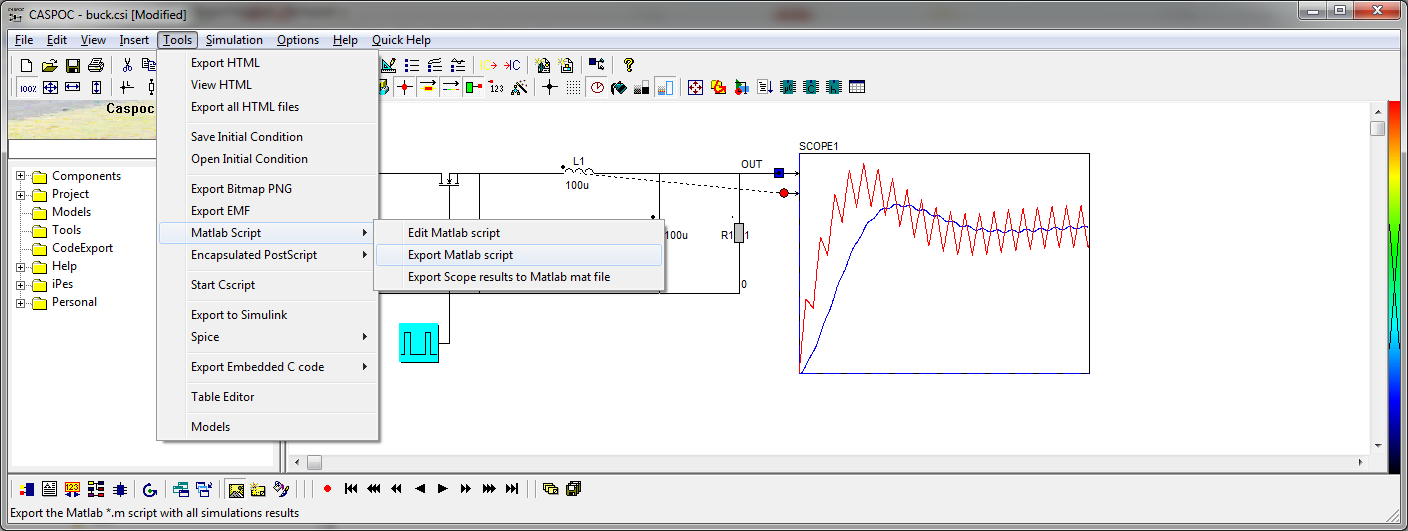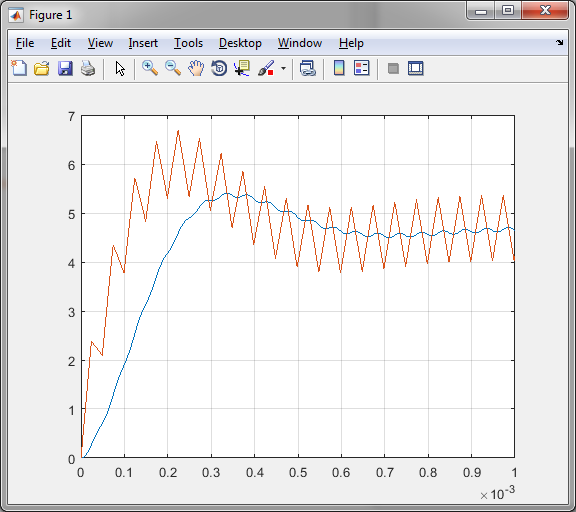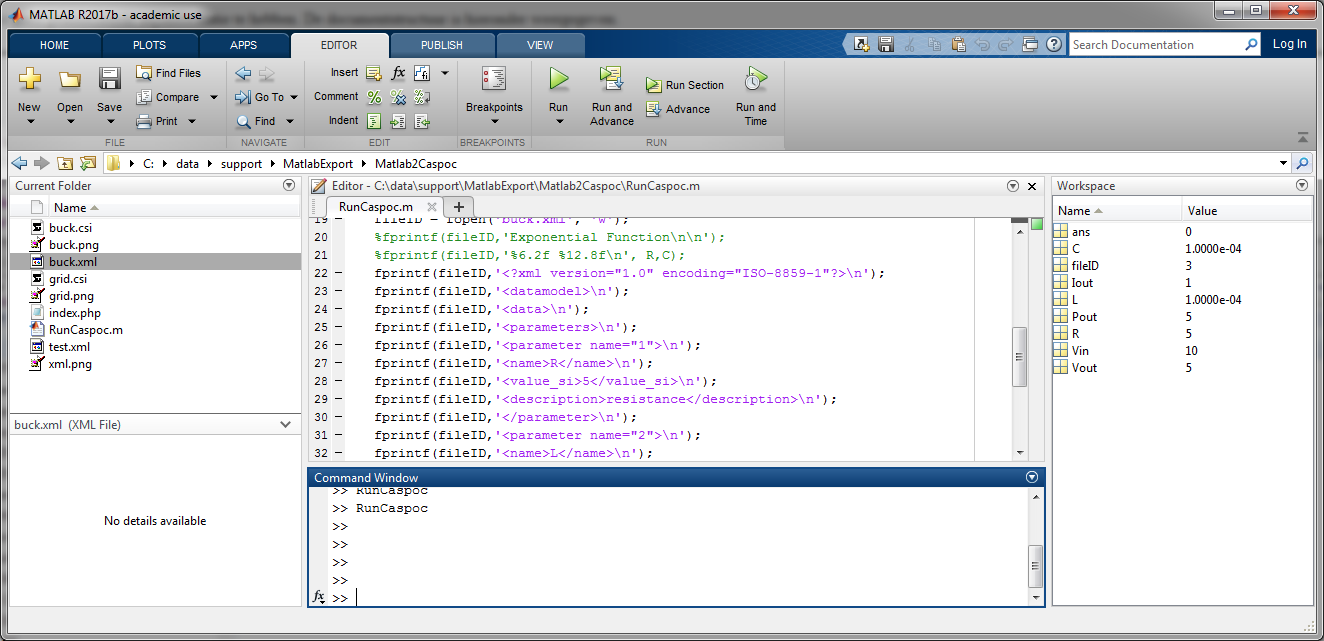• Introduction
• What is in this manual
• What is Caspoc
• User interface
• Introduction
• Starting
• Simulation
• Editing
• Viewing and printing
• Getting Started
• Basic editing
• Simulation in the time domain
• Basic User Interface Topics
• Editing
• Simulation
• Viewing
• Library
• Reports
• Project management
• Circuit and Block Diagram Components
• Introduction
• Cscript and user defined functions
• Component parameters
• Modeling Topics
• Introduction
• Power Electronics
• Semiconductors
• Electrical Machines
• Electrical drives
• Power Systems
• Mechanical Systems
• Thermal Systems
• Magnetic Circuits
• Green Energy
• Coupling to FEM
• Experimenter
• Analog hardware description language
• Embedded C code Export
• Coupling to Spice
• Small Signal Analysis
• Matlab coupling
• Tips and tricks
• Appendices

## Caspoc to Matlab export introduction.

You can export the simulation results from Caspoc into Matlab. This is advantageous when modeling power electronics, since Caspoc is better suited for modeling switching circuits, especially power electronics.

There are two methods for exporting results from Caspoc to Matlab. The m script and the mat file export method. The mat file method is preferred, since the size of the exported mat file is smaller than the m script.

• *.m script
The results from the simulation stored inside the scopes are exported into a matlab m script. For large amounts of data, the file size can be large, however, you have direct access to all data.
• *.mat file
The results from the simulation stored inside the scopes are exported into a matlab mat file. For large amounts of data, this method is preferred.

In the following two examples we are going to export the simulations results from the scope to Matlab.After finishing the simulation we can choose to export the m script or the mat file.By adding the plot() command in matlab, you can plot the contents in a figure.The last tutorial shows how to export data from Matlab to Caspoc.